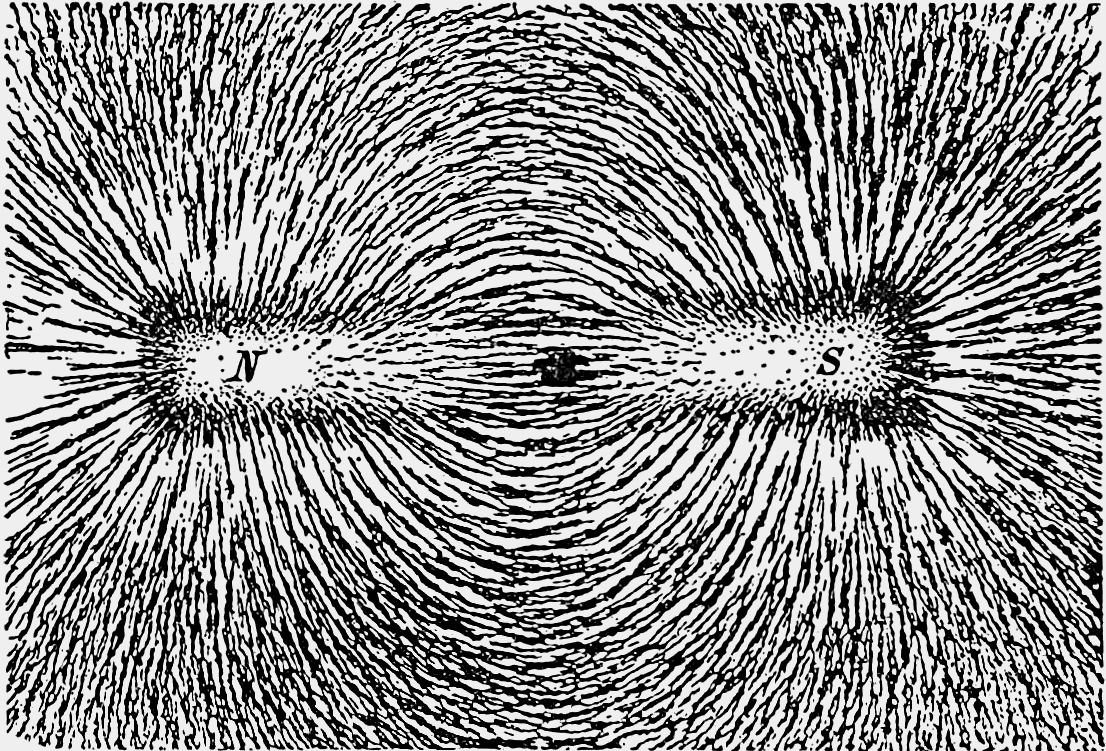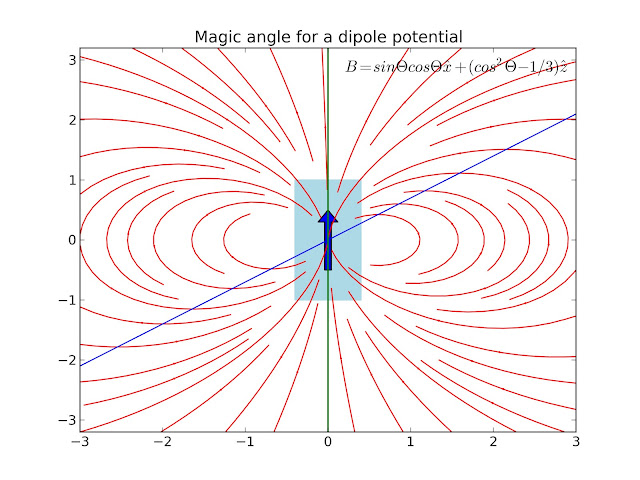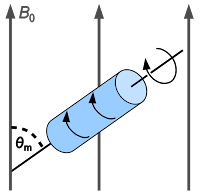## Thursday, 23 May 2013

### Fitting the noise

One of the new additions to PyTrA is Markov Chain Monte Carlo model checking. To begin I will go through Bayesian statistics, coding this up in python, using the pymc library and comparing this with normal fitting techniques.

## Bayesian Statistics

The technique relies on Baye's theorem. Most people know about simple probability theorem. All probabilities must add to one and you can model simple systems using this. However, what if we have some additional evidence either in the form of data or some prior knowledge about the model? We then can use Baye's theorem to describe this. Consider some data $D$ and a model $\theta$. The probability of the model given the data $P(\theta|D)$ is given by:

$P(\theta|D)=\frac{P(D|\theta)P(\theta)}{P(D)}$
• $P(\theta|D)$ is the Posterior distribution the probablity of the model parameter given the data which is what we want to find out.
• $P(D|\theta)$ is the Likelihood estimate the probability of the data given the parameter or the compatibility of the data with the given parameters.
• $P(\theta)$ is the Prior distribution the probability of the parameters apart from the data (for example we know that the pulse width of the pulse is not going to be negative).
• $P(D)$ is the probability of the data and is the normalising factor. As we consider ratios of probability densities this will cancel out using MCMC.
One of the applications of Bayesian inference is the scientific method. Baye's theorem guides the certainty of the models based on new observations and experiments.

You could try and integrate over all possible values for the parameters in your model, however with multidimensional data this no longer becomes possible within a reasonable timescale. We can use Monte Carlo integration which uses random numbers to form and estimate for the density of the parameters. Briefly, a Markov Chain is a time series which depends only on the previous step.

Metropolis and Hastings came up with a method to perform Monte Carlo integration using a Markov Chain to get $P(D|\theta)$.
1. Start with an initial point $\theta^{0}$.
2. Pick a new point $\theta^{*}$  according to some jumping distribution $q(\theta^{1},\theta^{2})$.
3. Calculate the ratio of the density after and before (acceptance ratio $\alpha$). $\alpha = \frac{p(\theta^{*})}{p(\theta^{t-1})}$.
4. If the jump increases the density $\alpha$ greater than one accept candidate point otherwise choose a random number $u$ between 0 and 1. If $alpha$ greater than $u$, accept the candidate point.
Hastings generalised the acceptance ratio to take into account unsymmetrical transitions using a transition probability function $q(\theta^{1},\theta^{2})$.

Define our model

We with try and model the noise of the data and the parameters. Let us choose the exponential decay as an example $Aexp(-t/\tau)$. If we subtract the model from the data we assume that data is described by a Gaussian or normal distribution
$P(t; \sigma, \tau, A|D) \propto P(D|t; \sigma, \tau, A)P(t; \sigma, \tau, A)$
$P(D|t; \sigma, \tau, A)=Norm(\mu=(\sum_{i}A exp(-t_{i}/\tau)-D_{i}),\sigma^{2})$
The priors $P(\sigma, \tau, A)$ we will take as uniform distribution  for the parameters that they are positive. For non-linear regression it is best to use a uniform distribution that does not limit the chain but uses prior knowledge responsibly.

## Code it up in Python

Initially just a simple MCMC sampler
import numpy as np
import matplotlib.pylab as plt
import numpy.random as ran

#Setting the random generator seed
ran.seed(6041510)

#Functions
def decay(t,tau,A):
return A*np.exp(-(t)/tau)

def loggaussdis(d,f,sig):
return np.sum(-(d-f)**2/sig**2)

def randchange(var,d):
return (var+ran.uniform(-d,d))

#Generate test data
size = 1000
tdata = np.linspace(0, 100, size)
tau = 20
A = 0.03
sig = 0.002

#Fitting using leastsquares fitting

#Monte Carlo scheme
simsize = 50000 #Iterations
tau_d = 0.5 #magnitude of change for tau
A_d = 0.01 #magnitude of change for A
tau = 19 #Initial value for tau
A = 0.025 #Initial value for A

#Results array for saving values
Res = np.ones((simsize,3))

for i in range(simsize):
#Calculate density of current point

#Change parameters
tau1 = randchange(tau,tau_d)
A1 = randchange(A,A_d)

#Calculate new density

#Due to densities being Gaussian this becomes subtraction
#This is our alpha
logB = dist2-dist1

#enforce the prior that both must be possitive.
if tau1<=0 or A1<=0:
tau = tau
A = A
else:
#If log B greater than 0 then accept change
if logB>=0:
tau = tau1
A = A1
else:
# if closer to one then more likely to be a good step
if ran.rand()<=np.exp(logB):
tau = tau1
A = A1

#print to results matrix
Res[i,0] = logB
Res[i,1] = tau
Res[i,2] = A

#Plotting of results

step = np.linspace(0, simsize, simsize)

plt.figure()
plt.title("sample data")

plt.figure()

plt.subplot(221)
plt.hist(Res[:,1],50)

plt.subplot(222)
plt.hist(Res[:,2],50)

plt.subplot(223)
plt.plot(step,Res[:,1])
plt.title("tau")

plt.subplot(224)
plt.plot(step,Res[:,2])
plt.title("A")

plt.show()
Data of the exponential decay with overlaid noiseMCMC simulation: bottom plots are the values of the parameters at the different iteration steps. The top graphs are the histograms of the distribution of the parameters.

This is made easier by the pymc library for python. We can also try and sample sigma and fit the noise as well as the model parameters.
Code for pymc
#Implementing it in pymc
import pymc

#sigma value for error out into dictionary

d={}
sig = pymc.Uniform('sig', 0.0, 0.005, value=0.0018)
d['sig'] = sig
A = pymc.Uniform('A', 0.02, 0.04, value= 0.025)
d['A'] = A
tau = pymc.Uniform('tau', 10.0, 30.0, value= 20.0)
d['tau'] = tau

#model defined as deterministic
@pymc.deterministic(plot=False)
def f(tdata=tdata,tau=tau,A=A):
return A*np.exp(-(tdata)/tau)

#likelihood Normal centred on the data with an error 1/sig^2.
d['y'] = pymc.Normal('y', mu=f, tau=1/sig/sig, value=Adata, observed=True)

R = pymc.MCMC(d)    #  build the model
R.sample(10000)
pymc.Matplot.plot(R)
plt.show()

You then get plots of the different parameters.
Plots for chain of A: top left if the value of the parameter at different iteration steps, bottom left autocorrelation plot, right is the histogram for the parameters.
Plots for the chain sigma the error of the noise we are modelling.
Plots for the chain tau of the time decay of the exponenital.

Now we can define a number of iterations to discard the burn in number. The autocorrelation is also large this can be reduced by tuning the acceptance criteria or by removing every nth number of traces. We will use burn=1000 and thin=5 so we will discard the first 1000 iterations and only use every fifth trace.
Using the method stats() you can get the mean and standard deviation.
print 'A','mean =',R.stats()['A']['mean'],'stdev =',R.stats()['A']['standard deviation']
print 'sig','mean =',R.stats()['sig']['mean'],'stdev =',R.stats()['sig']['standard deviation']
print 'tau','mean =',R.stats()['tau']['mean'],'stdev =',R.stats()['tau']['standard deviation']

A mean = 0.0299488396915 stdev = 0.000293780592428
sig mean = 0.00202956207822 stdev = 4.62119652347e-05
tau mean = 19.7427378282 stdev = 0.274207478109

Let us compare this with a simple least squares fit which you can use to deterministically minimise the $\chi^{2}$.
import scipy
vals, cov = scipy.optimize.curve_fit(decay, tdata, Adata)
print 'tau','mean =',vals,'stdev =',cov[0,0]
print 'A','mean =',vals,'stdev =',cov[1,1]

tau mean = 19.7517453814 stdev = 0.0724630777926
A mean = 0.0299249150895 stdev = 8.25426324691e-08

The MCMC method gave values very close to the true value.  It was also able to recover the noise from the data, not only its values but also its structure.

## References

Gamerman, D. and Lopes, H. F., "Markov Chain Monte Carlo: Stochastic Simulation for Bayesian Inference," 2006, NW, Chapman & Hall/CRC

## Wednesday, 1 May 2013

### The Magic Angle

I was looking at some electrodynamics before I go into modelling waveguides. At the same time I was looking into solid state NMR and also polarisation conditions in our laser system at the lab; both these techniques make use of the magic angle in order to remove dipole effects.

## What is a dipole?

Dipoles appear in many different parts of nature. The simplest to think about is a magnet. By putting iron filing around a bar magnet the so-called field lines (lines of equal potential/magnetism) can be seen.Bar magnet under paper with iron filing showing the field lines
As a convention we draw lines with arrows from the north to the south pole. The arrows denote the vector (vectors have direction and magnitude i.e. velocity).Representation of a dipole
Dipoles also appear between charged particles such as a positive nucleus of an atom and the electron, where the arrows go from positive to negative by convention.

## Physics of a dipole

A good explanation of how you can work out the field from a dipole can be seen here. Briefly, you can imagine two charged particles a set distance apart. You can work out the fields from simple electrostatics as the sum of the electric field from two points' charges and then as they come close together you end up with a point source dipole. This also works for magnetism, however as Maxwell's equations tell us magnetic flux cannot be created or come from a source so there is no divergence in the field. Mathematically it looks like this:

$\nabla \cdot B = 0$

But basically it means that if I were to draw a box around the dipole, the number of field lines going in (flux in) would equal the number of field lines going out (flux out).

Thinking about two nuclei close to each other, there will be an addition or subtraction to the overall magnetic field from the magnetic field of the other nuclei. In order to remove this we can look at the z term of the magnetic field (in this case along the axis of the dipole).

$B_{z}=\frac{|\mu|}{r^{3}}(3cos^{2}\theta -1)$

We are wanting to see when this contribution goes to zero. Setting this to zero, we see that when the z component of the magnetic field is zero, giving an angle of 54.7 degrees. This is what is called the magic angle.

## Modelling a dipole

To illustrate this I have a small script I wrote that plots the field lines from a magnet and then integrates a path (in green) where a particle would travel if influenced by the field. The line (in blue) drawn is at 54.7 degrees.

The plot is a quiver plot with the arrows indicating the field of the magnetic field at that point. You can see where the blue line at 54.7 degrees intersects the arrows that the z component is zero.

from pylab import *
from numpy import ma
import numpy as np
import matplotlib.pyplot as plt
import matplotlib
from matplotlib.collections import PatchCollection
import matplotlib.patches as mpatches
from scipy.integrate import odeint

#Size of simulation box
xmax=3.0
xmin=-xmax
NX=19
zmax=3.2
zmin=-zmax
NZ=19

# Setting up the grid
x=linspace(xmin,xmax,NX)
z=linspace(zmin,zmax,NZ)
X, Z = meshgrid(x, z)

#Function that describes the vector field for the integrator
def f(Y,t):
X, Z = Y
R = np.sqrt(X**2 + Z**2)
return((0.5*(3*X*Z/R**5)),(0.5*(3*Z**2/R**5 - 1/R**3)))

#Applying the field to the gridded values
R = np.sqrt(X**2 + Z**2)
Bx = 0.5*(3*X*Z/R**5)
Bz = 0.5*(3*Z**2/R**5 - 1/R**3)

#Had to mask some values so that you don't get large arrows right in the middle
M = zeros((X.shape,Z.shape), dtype='bool')
a, b = (NX/2), (NZ/2)
r=3.1

E,W = np.ogrid[-a:NX-a, -b:NZ-b]
print E.shape, W.shape,
mask = E*E + W*W <= r*r

#Magic angle line
line = 0.7*x
up = z*0

#Setting up plot
fig = plt.figure()

#Integrating paths
for z20 in [-1.0, 1.0]:
tspan = np.linspace(0, 62, 100)
z0 = [z20, 0.9]
zs = odeint(f, z0, tspan)
plt.plot(zs[:,0],zs[:,1], 'g-') # path

#Box and arrow
arr1 = matplotlib.patches.Arrow(0, -0.5, 0, 1, width=0.4)
rect1 = matplotlib.patches.Rectangle((-0.4,-1),0.8, 2, color='lightblue')

#Plotting
plot(x,line,color='blue')
plot(up,z,color='red')
a = title("Magic angle for a dipole")
plt.text(0, 2, "$B=sin \Theta cos \Theta \hat{x} + (cos^{2} \Theta - 1/3)\hat{z}$",size='large')
savefig('dipole.png',dpi=300)


I also tried out the new streamlines plot in matplotlib.Field lines of a magnet (red) with line drawn at the magic angle (blue) seen intersecting the field lines exactly where the z component (upwards component) of the field is zero.

#Setting up new range for streamlines
xmax=3.0
xmin=-xmax
NX=100
zmax=3.2
zmin=-zmax
NZ=100

x=linspace(xmin,xmax,NX)
z=linspace(zmin,zmax,NZ)
X, Z = meshgrid(x, z)

#Function for the vector field
R = np.sqrt(X**2 + Z**2)
Bx = 0.5*(3*X*Z/R**5)
Bz = 0.5*(3*Z**2/R**5 - 1/R**3)

fig = plt.figure()

#New streamplot in matplotlib
QS = streamplot(X,Z,Bx,Bz,density=[1.3,1.3],linewidth=1,color='red',minlength=0.3)

arr1 = matplotlib.patches.Arrow(0, -0.5, 0, 1, width=0.4)
rect1 = matplotlib.patches.Rectangle((-0.4,-1),0.8, 2, color='lightblue')

plot(x,line,color='blue',)
plot(up,z,color='green',linewidth=1.5)
a = title("Magic angle for a dipole potential")
plt.text(0.2, 2.8, "$B=sin \Theta cos \Theta \hat{x} + (cos^{2} \Theta - 1/3)\hat{z}$",size='large')

savefig('dipole_streamlines.png',dpi=300)


We we see is that at the magic angle we remove all of the effects of the magnetic field in the z direction on the particle.

In NMR they use rotors that orientate the crystal at the magic angle relative to the magnet field. With the spinning removing any directional effects. This lead to a nice liquid-like NMR peak.http://en.wikipedia.org/wiki/Magic_angle_spinning

In the Photon Factory we use a pump-probe technique to excite molecules and then see how they decay with time. We polarise the probe light (polarisation is the direction of the electric field) so that it is at the magic angle relative to the pump. This removes any polarisation dependencies from the two incoming beams with the sample (interesting measurements changing the polarisation of the pump and probe. This anisotropy can be used to infer structure of the molecule and other interesting properties).Menzel R., "Photonics: Linear and Nonlinear Interactions of Laser Light and Matter"
References

http://bulldog2.redlands.edu/facultyfolder/deweerd/tutorials/Tutorial-QuiverPlot.pdf
Field of a small dipole
http://www.physicsinsights.org/dipole_field_1.html